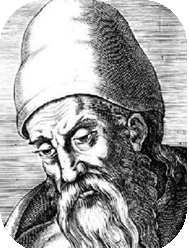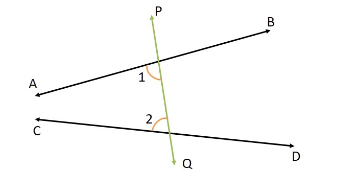## EuclidEuclid was a Greek mathematician, often referred to as the “founder of geometry”.

His Elements is one of the most influential works in the history of mathematics. In the Elements, Euclid deduced the theorems of what is now called Euclidean geometry from a small set of axioms.

He was likely born c. 325 BC. The place and circumstances of both his birth and death are unknown and have only been estimated roughly relative to other people mentioned with him.

Euclid died c. 270 BC, presumably in Alexandria

1. Any two points can be joined by a straight line segment.
2. Any straight line segment can be extended indefinitely in a straight line.
3. Given any straight line segment, a circle can be drawn having the segment as radius and one endpoint as center.
4. All right angles are congruent.
5. If two lines are drawn which intersect a third in such a way that the sum of the inner angles on one side is less than two right angles (180°), then the two lines must intersect each other on that side if extended far enough. Euclid’s fifth postulate is equivalent to what is known as the Parallel Postulate.